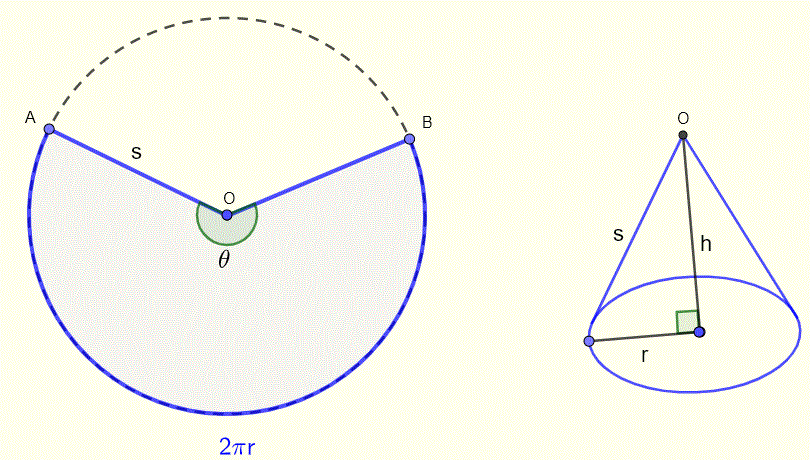# Make a Cone from a Sector Calculator

To make a cone, we start with a sector of central angle θ and radius s, we then joint points A and B letting point O move upward untill OA and OB are coincident. The radius s of the sector is equal to the slant height s of the cone.## Calculator to Angle $$\theta$$ and Radius $$s$$ of the Sector to Make a Cone

Enter the radius r of the base and height h of the cone as positive real numbers and press "Calculate". The outputs are the angle $$\theta$$ in degrees and the radius s of the sector needed to make the cone.
 r = 6 h = 8 $$\theta$$ = $$^{\circ}$$ s =

## Formulas for the Angle and Radius of the Sector

Let us suppose we want to make a cone with radius of the circular base r a height h. For the design of the cone, we need to find formulas for $$\theta$$ and $$s$$ of the sector in terms of $$r$$ and $$h$$ of the cone.
The length of the arc of the sector AB is given by
$$\theta s$$ , where $$\theta$$ is in radians.
The circumference of the circular base of the cone is given by
$$2\pi r$$

Since we join segment OA and OB to make the cone, the length of the arc AB of the sector is equal to the circumference of the circular base of the cone, hence
$$\theta s = 2\pi r$$
Solve the above for $$\theta$$
$$\theta = \dfrac{2\pi r}{s}$$
Use the Pyhtagoren theorem on the cone (right side of diagram), we
$$s^2 = r^2 + h^2$$
Solve for s
$$s = \sqrt{r^2 + h^2}$$
Substitute s in the formula for $$\theta$$
$$\theta = \dfrac{2\pi r}{\sqrt{r^2 + h^2}}$$ , in radians
Convert $$\theta$$ in degrees
$$\theta = \dfrac{2\pi r}{\sqrt{r^2 + h^2}} \dfrac{180}{\pi} = 180 \dfrac{2 r}{\sqrt{r^2 + h^2}}$$ , in degrees

Example
Find the angle $$\theta$$ of the sector and its radius s in order to make a cone of heightt h = 8 cm and a radius r = 6 cm.
Solution
$$s = \sqrt{r^2+h^2} = \sqrt{6^2+8^2} = 10 cm$$
$$\theta = 180 \dfrac{2 r}{\sqrt{r^2 + h^2}} = 180 \dfrac{2 \times 6}{\sqrt{6^2 + 8^2}} = 216^{\circ}$$